# Wasserstein Barycenters using Mosek¶

Wasserstein Distance is a way to measure the distance between two probabilty distributions. It allows to summarize, compare, match and reduce the dimensionality of the emprical probability measures to carry out some machine learning fundamentals.

Wasserstein distance of order $p$ between two probabilty measures $\mu$ and $\upsilon$ in $P(\Omega)$ is defined as:

$$W_p(\mu,\upsilon) \overset{\underset{\mathrm{def}}{}}{=} \bigg( \underset{\pi \in \Pi{(\mu, \upsilon)}}{\mbox{inf}} \int_{\Omega^2} D(X_i,Y_j)^p d\pi(x,y)\bigg)^{1/p}$$
where $\Pi(\mu, \upsilon)$ is the set of all probability measures on $\Omega^2$

If the distributions are discrete $W_p(\mu,\upsilon)$ is equilavent to the objective of the following LP model:

$$\mbox{minimize} \quad \sum_{i=1}\sum_{j=1} D(X_i,Y_j)^p\pi_{ij}$$
$$\mbox{st.} \quad \sum_{j=1} \pi_{ij} = \mu_i , \quad i = 1,2,..n$$
$$\quad \sum_{i=1} \pi_{ij} = \upsilon_j, \quad j = 1,2,..m$$
$$\pi_{ij} \geq 0, \quad \forall_{i,j}$$
where $D(X_i,Y_j)$ is the distance function on $\Omega$.

There are more efficient ways to approximate this metric but LP approach will be applied in order to compare the performance and modeling structure of Fusion, Pyomo and CVXPY.

## Wasserstein Barycenter¶

Wasserstein barycenter problem involves all Wasserstein distances from one to many measures. Given measures $\upsilon_1,\ldots,\upsilon_N$ we want to find the measure which minimizes the sum of distances to the given measures, that is solve the problem: $$\mbox{minimize}_\mu\sum_{i=1} \lambda_i W_p(\mu,\upsilon_i)$$ for some fixed system $\lambda_i$ of weights of distances to specific distributions that satisfies $$\sum_{i=1}\lambda_i = 1.$$ For simplicity uniform weights are used in this problem. Then the barycenters problem becomes: $$\mbox{minimize}_\mu \frac1N \sum_{i=1}^{N} W_p(\mu,\upsilon_i).$$

In this problem, Wasserstein Barycenter of One's are visualized using images with size $28x28$ using $20$ handwriten '1' digits from MNIST database http://yann.lecun.com/exdb/mnist/. Computations are carried out by Intel(R) Xeon(R) CPU E5-2687W v4 @ 3.00GHz processor. Similar experiments are performed by Cuturi and Doucet in http://proceedings.mlr.press/v32/cuturi14.pdf.

In :
import struct
import numpy as np
%matplotlib inline
import matplotlib.pyplot as plt

#Define the number of images for the barycenter calculation
n=20

#Read the images from the file
with open(filename, 'rb') as f:
zero, data_type, dims = struct.unpack('>HBB', f.read(4))
shape = tuple(struct.unpack('>I', f.read(4)) for d in range(dims))

#Select the images
ones = data[labels == 1]
train_1 = ones[:n]

plt.figure(figsize=(20,10))
for i in range(10):
plt.subplot(2,5,i+1)
plt.imshow(ones[np.random.randint(0,ones.shape)])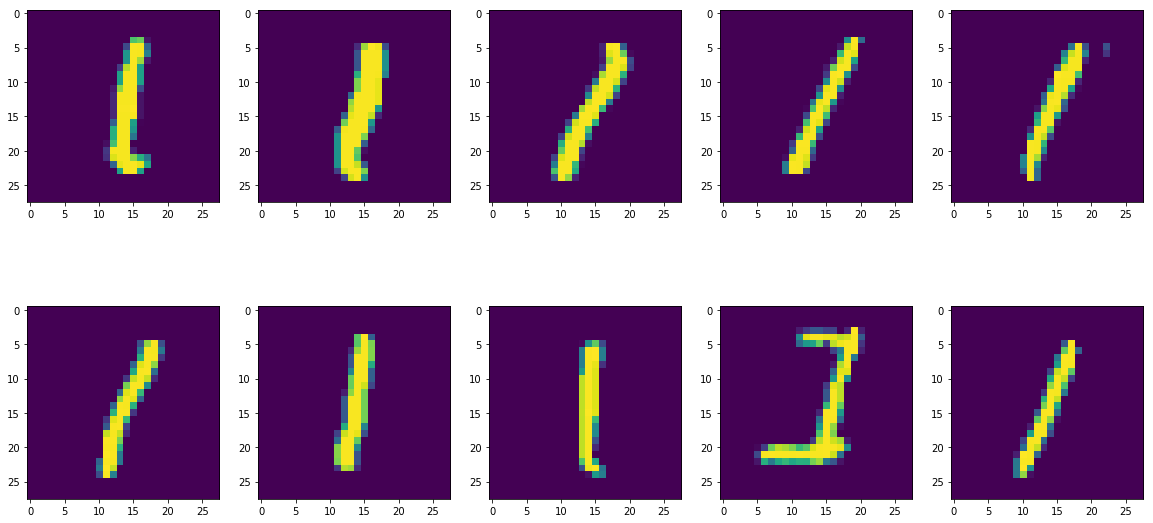## Barycenters using Mosek Fusion¶

The final barycenter problem is as follows. We choose $p=2$.

$$\mbox{minimize} \quad \frac1N \sum_{i,j,k}^{N} D(X_i,Y_j)^2\pi_{ij}^k$$
$$\mbox{st.} \quad \sum_{j=1} \pi_{ij}^{k} = \mu_i, \quad \forall_{k,i} \quad (1)$$
$$\quad \sum_{i=1} \pi_{ij}^{k} = \upsilon_j^{k}, \quad \forall_{k,j} \quad (2)$$
$$\pi_{ij}^{k} \geq 0 \quad \forall_{k,i,j}$$
where $D(X_i,Y_j)$ is the Euclidean distance between pixels and $N$ is the number of samples.

In :
from mosek.fusion import *
import time
import sys
class Wasserstein_Fusion:

def __init__(self):
self.time = 0.0
self.M = Model('Wasserstein')
self.result = None

def single_pmf(self, data = None, img=False):

''' Takes a image or array of images and extracts the probabilty mass function'''

if not img:
v=[]
for image in data:
arr = np.asarray(image).ravel(order='K')
v.append(arr/np.sum(arr))
else:
v = np.asarray(data).ravel(order='K')
v = v/np.sum(v)
return v

def ms_distance(self, m ,n, constant=False):

''' Squared Euclidean distance calculation between the pixels '''

if constant:
d = np.ones((m,m))
else:
d = np.empty((m,m))
coor = []
for i in range(n):
for j in range(n):
coor.append(np.array([i,j]))
for i in range(m):
for j in range(m):
d[i][j] = np.linalg.norm(coor[i]-coor[j])**2
return d

def Wasserstein_Distance(self, bc ,data, img = False):

''' Calculation of wasserstein distance between a barycenter and an image by solving the minimization problem '''

v = np.array(self.single_pmf(data, img))
n = v.shape
d = self.ms_distance(n,data.shape)
with Model('Wasserstein') as M:
pi = M.variable('pi',[n,n], Domain.greaterThan(0.0))

M.constraint('c1' , Expr.sum(pi,0), Domain.equalsTo(v))
M.constraint('c2' , Expr.sum(pi,1), Domain.equalsTo(bc))

M.objective('Obj.' , ObjectiveSense.Minimize, Expr.dot(d, pi))

M.solve()
objective = M.primalObjValue()

return objective

def Wasserstein_BaryCenter(self,data):

M = self.M
start_time = time.time()
k = data.shape
v = np.array(self.single_pmf(data))
n = v.shape
d = self.ms_distance(n,data.shape)

mu = M.variable('Mu', n, Domain.greaterThan(0.0))
pi = (M.variable('Pi', [k,n,n] , Domain.greaterThan(0.0)))

#Constraint (1)
M.constraint('B', Expr.sub(Expr.sum(pi,1) , Var.repeat(mu,1,k).transpose()), Domain.equalsTo(0.0))
#Constraint (2)
M.constraint('C', Expr.sum(pi,2), Domain.equalsTo(v))

M.objective('Obj' , ObjectiveSense.Minimize, Expr.sum(Expr.mul(Expr.mul(Expr.reshape(pi.asExpr(), k, n*n) , d.ravel()), 1/k)))

M.setLogHandler(sys.stdout)
M.solve()
self.result = mu.level()
M.selectedSolution(SolutionType.Interior)
self.objective = M.primalObjValue()
self.time = time.time() - start_time

return mu.level()

def reset(self):
self.M = Model('Wasserstein')


In :
fusion_model = Wasserstein_Fusion()
f_bc = fusion_model.Wasserstein_BaryCenter(train_1)
print('\nTime Spent to solve problem with Fusion: \n {0}'.format(fusion_model.time))
print('Time Spent in solver: \n {0}'.format(fusion_model.M.getSolverDoubleInfo("optimizerTime")))
print('The average Wasserstein distance between digits and the barycenter: \n {0}'.format(fusion_model.objective))

Problem
Name                   : Wasserstein
Objective sense        : min
Type                   : LO (linear optimization problem)
Constraints            : 31360
Cones                  : 0
Scalar variables       : 12293905
Matrix variables       : 0
Integer variables      : 0

Optimizer started.
Presolve started.
Linear dependency checker started.
Linear dependency checker terminated.
Eliminator started.
Freed constraints in eliminator : 0
Eliminator terminated.
Eliminator - tries                  : 1                 time                   : 0.00
Lin. dep.  - tries                  : 1                 time                   : 0.35
Lin. dep.  - number                 : 19
Presolve terminated. Time: 5.70
GP based matrix reordering started.
GP based matrix reordering terminated.
Problem
Name                   : Wasserstein
Objective sense        : min
Type                   : LO (linear optimization problem)
Constraints            : 31360
Cones                  : 0
Scalar variables       : 12293905
Matrix variables       : 0
Integer variables      : 0

Optimizer  - solved problem         : the primal
Optimizer  - Constraints            : 17440
Optimizer  - Cones                  : 0
Optimizer  - Scalar variables       : 1395520           conic                  : 0
Optimizer  - Semi-definite variables: 0                 scalarized             : 0
Factor     - setup time             : 16.44             dense det. time        : 0.21
Factor     - ML order time          : 0.04              GP order time          : 14.45
Factor     - nonzeros before factor : 1.55e+06          after factor           : 1.44e+07
Factor     - dense dim.             : 0                 flops                  : 1.64e+10
Factor     - GP saved nzs           : 1.75e+06          GP saved flops         : 3.15e+09
ITE PFEAS    DFEAS    GFEAS    PRSTATUS   POBJ              DOBJ              MU       TIME
0   5.5e+03  3.2e+02  8.3e+07  0.00e+00   1.182613040e+07   0.000000000e+00   4.9e+01  23.93
1   6.8e-01  4.0e-02  1.0e+04  -1.00e+00  1.120231068e+07   -1.638527125e+05  6.1e-03  24.96
2   5.8e-02  3.4e-03  8.8e+02  2.61e+01   1.558624710e+04   -2.985003979e+03  5.2e-04  26.57
3   4.5e-02  2.6e-03  6.8e+02  1.08e+01   3.256531514e+03   -6.820367762e+02  4.0e-04  27.67
4   4.2e-02  2.5e-03  6.4e+02  5.25e+00   2.407863248e+03   -4.995626420e+02  3.8e-04  28.62
5   3.8e-02  2.2e-03  5.7e+02  4.40e+00   1.571023848e+03   -3.171235705e+02  3.4e-04  29.52
6   3.3e-02  1.9e-03  5.0e+02  3.48e+00   1.104555995e+03   -2.125212567e+02  3.0e-04  30.36
7   2.9e-02  1.7e-03  4.3e+02  2.91e+00   7.928892307e+02   -1.415543053e+02  2.6e-04  31.30
8   1.2e-02  7.1e-04  1.8e+02  2.47e+00   2.252271180e+02   -1.976502285e+01  1.1e-04  32.37
9   5.2e-03  3.1e-04  7.9e+01  1.44e+00   9.137862868e+01   -2.802278257e+00  4.7e-05  33.33
10  1.2e-03  6.9e-05  1.8e+01  1.16e+00   2.223917710e+01   2.047778184e+00   1.1e-05  34.94
11  7.7e-04  4.5e-05  1.2e+01  1.04e+00   1.552925437e+01   2.419701859e+00   6.9e-06  35.99
12  3.9e-04  2.3e-05  5.9e+00  1.02e+00   9.411514139e+00   2.769092362e+00   3.5e-06  37.12
13  1.3e-04  8.1e-06  2.0e+00  1.01e+00   5.275173812e+00   3.000408169e+00   1.2e-06  38.69
14  5.5e-05  3.3e-06  8.2e-01  1.00e+00   3.990965725e+00   3.070294675e+00   4.9e-07  39.91
15  2.5e-05  1.5e-06  3.8e-01  1.00e+00   3.515474345e+00   3.095350472e+00   2.2e-07  40.99
16  1.2e-05  7.0e-07  1.8e-01  1.00e+00   3.304309669e+00   3.106260800e+00   1.1e-07  42.22
17  7.1e-06  4.3e-07  1.1e-01  1.00e+00   3.229689796e+00   3.109866447e+00   6.4e-08  43.16
18  3.1e-06  2.4e-07  4.8e-02  1.00e+00   3.165660320e+00   3.112133382e+00   2.8e-08  44.08
19  1.2e-06  9.5e-08  1.9e-02  1.00e+00   3.135045283e+00   3.113923931e+00   1.1e-08  45.09
20  2.5e-07  1.9e-08  3.9e-03  1.00e+00   3.119054866e+00   3.114731758e+00   2.3e-09  46.13
21  1.2e-09  9.1e-11  1.8e-05  1.00e+00   3.114878927e+00   3.114858639e+00   1.1e-11  47.44
22  3.4e-12  2.5e-13  4.8e-08  1.00e+00   3.114858825e+00   3.114858771e+00   2.8e-14  48.27
23  3.7e-13  5.7e-14  3.3e-13  1.00e+00   3.114858772e+00   3.114858772e+00   2.9e-18  49.09
Basis identification started.
Basis identification terminated. Time: 0.51
Optimizer terminated. Time: 53.52

Interior-point solution summary
Problem status  : PRIMAL_AND_DUAL_FEASIBLE
Solution status : OPTIMAL
Primal.  obj: 3.1148587718e+00    nrm: 1e+00    Viol.  con: 4e-13    var: 0e+00
Dual.    obj: 3.1148587718e+00    nrm: 2e+02    Viol.  con: 0e+00    var: 6e-14

Basic solution summary
Problem status  : PRIMAL_AND_DUAL_FEASIBLE
Solution status : OPTIMAL
Primal.  obj: 3.1148587718e+00    nrm: 1e+00    Viol.  con: 4e-17    var: 1e-06
Dual.    obj: 3.1148587718e+00    nrm: 2e+02    Viol.  con: 0e+00    var: 2e-09

Time Spent to solve problem with Fusion:
74.02755951881409
Time Spent in solver:
53.52407097816467
The average Wasserstein distance between digits and the barycenter:
3.1148587717997467

In :
fus_bc = np.reshape(f_bc,(28,28))
plt.imshow(fus_bc)
plt.title('Barycenter')
plt.show()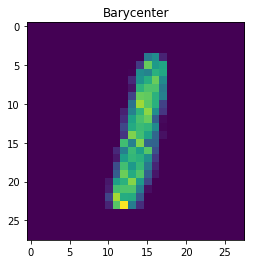## Modeling the same problem with Pyomo¶

The same problem is formulated using Pyomo and solved using Mosek. Unlike Fusion Pyomo requires rules and summations to formulate the problem.

In :
import pyomo.environ as pyo
import time
class Wasserstein_Pyomo:

def __init__(self):
self.time = 0.0
self.result = None
self._solver = 'mosek'
self.M = pyo.ConcreteModel()

def single_pmf(self, data = None, img=False):

''' Takes a image or array of images and extracts the probabilty mass function'''

if not img:
v=[]
for image in data:
arr = np.asarray(image).ravel(order='K')
v.append(arr/np.sum(arr))
else:
v = np.asarray(data).ravel(order='K')
v = v/np.sum(v)
return v

def ms_distance(self, m ,n, constant=False):

''' Squared Euclidean distance calculation between the pixels '''

if constant:
d = np.ones((m,m))
else:
d = np.empty((m,m))
coor = []
for i in range(n):
for j in range(n):
coor.append(np.array([i,j]))
for i in range(m):
for j in range(m):
d[i][j] = np.linalg.norm(coor[i]-coor[j])**2
return d

def Wasserstein_BaryCenter(self,data):

''' Calculation of wasserstein barycenter of given images by solving the minimization problem '''

M = self.M
k = data.shape
v = np.array(self.single_pmf(data))
n = v.shape
d = self.ms_distance(n,data.shape)

#Define indices
M.i = range(n)
M.j = range(n)
M.k = range(k)

M.pi = pyo.Var(M.k, M.i, M.j, domain = pyo.NonNegativeReals)
M.mu = pyo.Var(M.i, domain = pyo.NonNegativeReals)
M.t = pyo.Var(M.k, domain = pyo.NonNegativeReals)

M.obj = pyo.Objective(expr = sum(M.t[k] for k in M.k)/k, sense= pyo.minimize)

#Define constraint rules
def c3_rule(model, k, j): #Rule for Constraint (3)
return sum(model.pi[k,i,j] for i in model.i) == v[k][j]
def c2_rule(model, k, i): #Rule for Constraint (2)
return sum(model.pi[k,i,j] for j in model.j) == model.mu[i]
def c1_rule(model, k):    #Rule for Constraint (1)
return sum(d[i][j]*model.pi[k,i,j] for i in model.i for j in model.j) <= model.t[k]

M.c3 = pyo.Constraint(M.k, M.j , rule = c3_rule)
M.c2 = pyo.Constraint(M.k, M.i , rule = c2_rule)
M.c1 = pyo.Constraint(M.k, rule = c1_rule)

return M

def run(self,data):
start_time = time.time()
model = self.Wasserstein_BaryCenter(data)
opt = pyo.SolverFactory(self._solver)
self.result = opt.solve(model, tee = True)
self.time = time.time() - start_time
bc = []
[bc.append(model.mu[i]()) for i in range(data.shape*data.shape)]
return np.array(bc)

def reset(self):
self.M = pyo.ConcreteModel()


In :
pyomo_model = Wasserstein_Pyomo()
p_bc = pyomo_model.run(train_1)
print('\nTime spent to solve problem with Pyomo: \n {0}'.format(pyomo_model.time))
print('Time spent in solver: \n {0}'.format(pyomo_model.result.solver.wallclock_time))
print('The average Wasserstein distance between digits and the barycenter: \n {0}'.format(pyomo_model.M.obj()))

Problem
Name                   :
Objective sense        : min
Type                   : LO (linear optimization problem)
Constraints            : 31380
Cones                  : 0
Scalar variables       : 12293924
Matrix variables       : 0
Integer variables      : 0

Optimizer started.
Presolve started.
Linear dependency checker started.
Linear dependency checker terminated.
Eliminator started.
Freed constraints in eliminator : 0
Eliminator terminated.
Eliminator - tries                  : 1                 time                   : 0.00
Lin. dep.  - tries                  : 1                 time                   : 0.39
Lin. dep.  - number                 : 19
Presolve terminated. Time: 9.03
GP based matrix reordering started.
GP based matrix reordering terminated.
Problem
Name                   :
Objective sense        : min
Type                   : LO (linear optimization problem)
Constraints            : 31380
Cones                  : 0
Scalar variables       : 12293924
Matrix variables       : 0
Integer variables      : 0

Optimizer  - solved problem         : the primal
Optimizer  - Constraints            : 17440
Optimizer  - Cones                  : 0
Optimizer  - Scalar variables       : 1395520           conic                  : 0
Optimizer  - Semi-definite variables: 0                 scalarized             : 0
Factor     - setup time             : 16.13             dense det. time        : 0.22
Factor     - ML order time          : 0.04              GP order time          : 14.46
Factor     - nonzeros before factor : 1.55e+06          after factor           : 1.44e+07
Factor     - dense dim.             : 0                 flops                  : 1.64e+10
Factor     - GP saved nzs           : 1.75e+06          GP saved flops         : 3.15e+09
ITE PFEAS    DFEAS    GFEAS    PRSTATUS   POBJ              DOBJ              MU       TIME
0   5.5e+03  3.2e+02  8.3e+07  0.00e+00   1.182613040e+07   0.000000000e+00   4.9e+01  26.72
1   6.8e-01  4.0e-02  1.0e+04  -1.00e+00  1.120231111e+07   -1.638527136e+05  6.1e-03  27.80
2   5.8e-02  3.4e-03  8.8e+02  2.61e+01   1.558624799e+04   -2.985004062e+03  5.2e-04  29.37
3   4.5e-02  2.6e-03  6.8e+02  1.08e+01   3.256531589e+03   -6.820367758e+02  4.0e-04  30.39
4   4.2e-02  2.5e-03  6.4e+02  5.25e+00   2.407863322e+03   -4.995626462e+02  3.8e-04  31.33
5   3.8e-02  2.2e-03  5.7e+02  4.40e+00   1.571023931e+03   -3.171235815e+02  3.4e-04  32.18
6   3.3e-02  1.9e-03  5.0e+02  3.48e+00   1.104556065e+03   -2.125212675e+02  3.0e-04  33.06
7   2.9e-02  1.7e-03  4.3e+02  2.91e+00   7.928892926e+02   -1.415543160e+02  2.6e-04  34.05
8   1.2e-02  7.1e-04  1.8e+02  2.47e+00   2.252273003e+02   -1.976505585e+01  1.1e-04  35.13
9   5.2e-03  3.1e-04  7.9e+01  1.44e+00   9.137867985e+01   -2.802280352e+00  4.7e-05  36.09
10  1.2e-03  6.9e-05  1.8e+01  1.16e+00   2.223918431e+01   2.047776659e+00   1.1e-05  37.94
11  7.7e-04  4.5e-05  1.2e+01  1.04e+00   1.552926266e+01   2.419700435e+00   6.9e-06  38.99
12  3.9e-04  2.3e-05  5.9e+00  1.02e+00   9.413170641e+00   2.768991242e+00   3.5e-06  39.96
13  1.3e-04  8.1e-06  2.0e+00  1.01e+00   5.274879516e+00   3.000437008e+00   1.2e-06  41.68
14  5.5e-05  3.3e-06  8.2e-01  1.00e+00   3.991039163e+00   3.070294699e+00   4.9e-07  42.90
15  2.5e-05  1.5e-06  3.8e-01  1.00e+00   3.515553240e+00   3.095347848e+00   2.2e-07  44.03
16  1.2e-05  7.0e-07  1.8e-01  1.00e+00   3.304324846e+00   3.106260526e+00   1.1e-07  45.22
17  7.1e-06  4.3e-07  1.1e-01  1.00e+00   3.229734360e+00   3.109864486e+00   6.4e-08  46.19
18  3.1e-06  2.4e-07  4.8e-02  1.00e+00   3.165698228e+00   3.112133278e+00   2.8e-08  47.16
19  1.2e-06  9.4e-08  1.9e-02  1.00e+00   3.135045322e+00   3.113924772e+00   1.1e-08  48.29
20  2.5e-07  1.9e-08  3.9e-03  1.00e+00   3.119048189e+00   3.114732226e+00   2.3e-09  49.29
21  1.2e-09  9.1e-11  1.8e-05  1.00e+00   3.114878911e+00   3.114858640e+00   1.1e-11  50.64
22  3.6e-12  2.5e-13  4.7e-08  1.00e+00   3.114858824e+00   3.114858771e+00   2.8e-14  51.46
23  4.1e-13  7.1e-14  2.0e-12  1.00e+00   3.114858772e+00   3.114858772e+00   2.9e-18  52.27
Basis identification started.
Basis identification terminated. Time: 0.42
Optimizer terminated. Time: 58.87

Interior-point solution summary
Problem status  : PRIMAL_AND_DUAL_FEASIBLE
Solution status : OPTIMAL
Primal.  obj: 3.1148587718e+00    nrm: 6e+00    Viol.  con: 7e-13    var: 0e+00
Dual.    obj: 3.1148587718e+00    nrm: 2e+02    Viol.  con: 0e+00    var: 4e-14

Basic solution summary
Problem status  : PRIMAL_AND_DUAL_FEASIBLE
Solution status : OPTIMAL
Primal.  obj: 3.1148587718e+00    nrm: 6e+00    Viol.  con: 4e-17    var: 1e-06
Dual.    obj: 3.1148587718e+00    nrm: 2e+02    Viol.  con: 0e+00    var: 2e-09

Time spent to solve problem with Pyomo:
900.9914240837097
Time spent in solver:
58.86694002151489
The average Wasserstein distance between digits and the barycenter:
3.114858771795237

In :
pyo_bc = np.reshape(p_bc, (28,28))
#print('Visualization of the barycenter:')
plt.imshow(pyo_bc)
plt.title('Barycenter')
plt.show()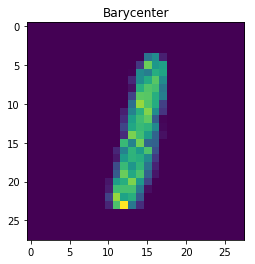### Modeling the same problem with CVXPY¶

In :
import cvxpy as cp
import time
class Wasserstein_CVXPY:

def __init__(self):
self.time = 0.0
self.result = None
self.prob = None

def single_pmf(self, data = None, img=False):

''' Takes a image or array of images and extracts the probabilty mass function'''

if not img:
v=[]
for image in data:
arr = np.asarray(image).ravel(order='K')
v.append(arr/np.sum(arr))
else:
v = np.asarray(data).ravel(order='K')
v = v/np.sum(v)
return v

def ms_distance(self, m ,n, constant=False):

''' Squared Euclidean distance calculation between the pixels '''

if constant:
d = np.ones((m,m))
else:
d = np.empty((m,m))
coor = []
for i in range(n):
for j in range(n):
coor.append(np.array([i,j]))
for i in range(m):
for j in range(m):
d[i][j] = np.linalg.norm(coor[i]-coor[j])**2
return d

def Wasserstein_Distance(self, bc ,data, img = False):

''' Calculation of wasserstein distance between a barycenter and an image by solving
the minimization problem '''

v = np.array(self.single_pmf(data, img))
n = v.shape
d = self.ms_distance(n,data.shape)

pi = cp.Variable((n,n), nonneg=True)
obj = cp.Minimize((np.ones(n).T @ cp.multiply(d,pi) @ np.ones(n)))

Cons=[]
Cons.append((np.ones(n) @ pi).T == bc)
Cons.append((pi @ np.ones(n)) == v)

prob = cp.Problem(obj, constraints= Cons)

return prob.solve(solver=cp.MOSEK, verbose = True)

def Wasserstein_BaryCenter(self,data):

''' Calculation of wasserstein barycenter of given images by solving the minimization problem '''

start_time = time.time()
k = data.shape
v = np.array(self.single_pmf(data))
n = v.shape
d = self.ms_distance(n,data.shape)

pi= []
t= []
mu = cp.Variable(n, nonneg = True)
for i in range(k):
pi.append(cp.Variable((n,n), nonneg = True))
t.append(cp.Variable(nonneg = True))

obj = cp.Minimize(np.sum(t)/k)

Cons=[]
for i in range(k):
Cons.append( t[i] >= np.ones(n).T @  cp.multiply(d,pi[i]) @ np.ones(n) ) #Constraint (1)
Cons.append( (np.ones(n) @ pi[i]).T == mu)                               #Constraint (2)
Cons.append( (pi[i] @ np.ones(n)) == v[i])                            #Constraint (3)

self.prob = cp.Problem(obj, constraints= Cons)
self.result = self.prob.solve(solver=cp.MOSEK,verbose = True)
self.time = time.time() - start_time

return mu.value

def reset(self):
self.prob = None
self.result = None

In :
cvxpy_model = Wasserstein_CVXPY()
result = cvxpy_model.Wasserstein_BaryCenter(train_1)
print('\nTime Spent to solve problem with CVXPY: \n {0}'.format(cvxpy_model.time))
print('Time Spent in solver: \n {0}'.format(cvxpy_model.prob.solver_stats.solve_time))
print('The average Wasserstein distance between digits and the barycenter: \n {0}'.format(cvxpy_model.result))

Problem
Name                   :
Objective sense        : min
Type                   : LO (linear optimization problem)
Constraints            : 12325304
Cones                  : 0
Scalar variables       : 12293924
Matrix variables       : 0
Integer variables      : 0

Optimizer started.
Presolve started.
Linear dependency checker started.
Linear dependency checker terminated.
Eliminator started.
Freed constraints in eliminator : 0
Eliminator terminated.
Eliminator - tries                  : 1                 time                   : 0.00
Lin. dep.  - tries                  : 1                 time                   : 2.55
Lin. dep.  - number                 : 19
Presolve terminated. Time: 15.54
GP based matrix reordering started.
GP based matrix reordering terminated.
Problem
Name                   :
Objective sense        : min
Type                   : LO (linear optimization problem)
Constraints            : 12325304
Cones                  : 0
Scalar variables       : 12293924
Matrix variables       : 0
Integer variables      : 0

Optimizer  - solved problem         : the primal
Optimizer  - Constraints            : 17440
Optimizer  - Cones                  : 0
Optimizer  - Scalar variables       : 1395520           conic                  : 0
Optimizer  - Semi-definite variables: 0                 scalarized             : 0
Factor     - setup time             : 16.43             dense det. time        : 0.21
Factor     - ML order time          : 0.04              GP order time          : 14.57
Factor     - nonzeros before factor : 1.55e+06          after factor           : 1.44e+07
Factor     - dense dim.             : 0                 flops                  : 1.64e+10
Factor     - GP saved nzs           : 1.75e+06          GP saved flops         : 3.15e+09
ITE PFEAS    DFEAS    GFEAS    PRSTATUS   POBJ              DOBJ              MU       TIME
0   5.5e+03  3.2e+02  8.3e+07  0.00e+00   1.182613040e+07   0.000000000e+00   4.9e+01  34.19
1   6.8e-01  4.0e-02  1.0e+04  -1.00e+00  1.120231112e+07   -1.638527133e+05  6.1e-03  35.19
2   5.8e-02  3.4e-03  8.8e+02  2.61e+01   1.558624808e+04   -2.985004070e+03  5.2e-04  36.64
3   4.5e-02  2.6e-03  6.8e+02  1.08e+01   3.256531589e+03   -6.820367740e+02  4.0e-04  37.60
4   4.2e-02  2.5e-03  6.4e+02  5.25e+00   2.407863324e+03   -4.995626454e+02  3.8e-04  38.57
5   3.8e-02  2.2e-03  5.7e+02  4.40e+00   1.571023937e+03   -3.171235819e+02  3.4e-04  39.50
6   3.3e-02  1.9e-03  5.0e+02  3.48e+00   1.104556071e+03   -2.125212684e+02  3.0e-04  40.49
7   2.9e-02  1.7e-03  4.3e+02  2.91e+00   7.928892985e+02   -1.415543170e+02  2.6e-04  41.34
8   1.2e-02  7.1e-04  1.8e+02  2.47e+00   2.252273014e+02   -1.976505604e+01  1.1e-04  42.35
9   5.2e-03  3.1e-04  7.9e+01  1.44e+00   9.137868017e+01   -2.802280320e+00  4.7e-05  43.28
10  1.2e-03  6.9e-05  1.8e+01  1.16e+00   2.223918434e+01   2.047776637e+00   1.1e-05  44.86
11  7.7e-04  4.5e-05  1.2e+01  1.04e+00   1.552926278e+01   2.419700413e+00   6.9e-06  45.89
12  3.9e-04  2.3e-05  5.9e+00  1.02e+00   9.413192190e+00   2.768989925e+00   3.5e-06  46.88
13  1.3e-04  8.1e-06  2.0e+00  1.01e+00   5.274875679e+00   3.000437376e+00   1.2e-06  48.55
14  5.5e-05  3.3e-06  8.2e-01  1.00e+00   3.991040086e+00   3.070294698e+00   4.9e-07  49.88
15  2.5e-05  1.5e-06  3.8e-01  1.00e+00   3.515554299e+00   3.095347811e+00   2.2e-07  50.96
16  1.2e-05  7.0e-07  1.8e-01  1.00e+00   3.304325086e+00   3.106260520e+00   1.1e-07  52.26
17  7.1e-06  4.3e-07  1.1e-01  1.00e+00   3.229734970e+00   3.109864458e+00   6.4e-08  53.26
18  3.1e-06  2.4e-07  4.8e-02  1.00e+00   3.165698749e+00   3.112133276e+00   2.8e-08  54.22
19  1.2e-06  9.4e-08  1.9e-02  1.00e+00   3.135045328e+00   3.113924783e+00   1.1e-08  55.44
20  2.5e-07  1.9e-08  3.9e-03  1.00e+00   3.119048079e+00   3.114732233e+00   2.3e-09  56.55
21  1.2e-09  9.1e-11  1.8e-05  1.00e+00   3.114878914e+00   3.114858640e+00   1.1e-11  57.87
22  3.5e-12  2.5e-13  4.7e-08  1.00e+00   3.114858824e+00   3.114858771e+00   2.8e-14  58.71
23  4.6e-13  5.7e-14  2.8e-14  1.00e+00   3.114858772e+00   3.114858772e+00   2.9e-18  59.58
Basis identification started.
Basis identification terminated. Time: 0.40
Optimizer terminated. Time: 68.77

Interior-point solution summary
Problem status  : PRIMAL_AND_DUAL_FEASIBLE
Solution status : OPTIMAL
Primal.  obj: 3.1148587718e+00    nrm: 6e+00    Viol.  con: 3e-12    var: 0e+00
Dual.    obj: 3.1148587718e+00    nrm: 2e+02    Viol.  con: 9e-16    var: 6e-14

Basic solution summary
Problem status  : PRIMAL_AND_DUAL_FEASIBLE
Solution status : OPTIMAL
Primal.  obj: 3.1148587718e+00    nrm: 6e+00    Viol.  con: 1e-06    var: 0e+00
Dual.    obj: 3.1148587718e+00    nrm: 2e+02    Viol.  con: 2e-09    var: 2e-09

Time Spent to solve problem with CVXPY:
122.6916720867157
Time Spent in solver:
68.76518487930298
The average Wasserstein distance between digits and the barycenter:
3.1148587717983913

In :
plt.imshow(np.reshape(result.squeeze(), (28,28)))
plt.title('Barycenter')
plt.show()Also, when the log data from Mosek is investigated the number of variables are same for each modeling language. However, while Pyomo and Fusion having same number of constraints CVXPY has a lot of extra constraints that resulted from nonnegativity of the variables.

## Comparison of Modeling Languages¶

### Time to solve the problem¶

In :
print('Total Time:')
print('Fusion: {0}'.format(fusion_model.time))
print('Pyomo : {0}'.format(pyomo_model.time))
print('CVXPY : {0}'.format(cvxpy_model.time))
print('\nTime spent in the solver:')
print('Fusion: {0}'.format(fusion_model.M.getSolverDoubleInfo("optimizerTime")))
print('Pyomo : {0}'.format(pyomo_model.result.solver.wallclock_time))
print('CVXPY : {0}'.format(cvxpy_model.prob.solver_stats.solve_time))

Total Time:
Fusion: 74.02755951881409
Pyomo : 900.9914240837097
CVXPY : 122.6916720867157

Time spent in the solver:
Fusion: 53.52407097816467
Pyomo : 58.86694002151489
CVXPY : 68.76518487930298

In :
plt.style.use('seaborn')
plt.figure(figsize=(10,4))

total_t = [fusion_model.time, pyomo_model.time, cvxpy_model.time]
solver_t = [fusion_model.M.getSolverDoubleInfo("optimizerTime"), pyomo_model.result.solver.wallclock_time, cvxpy_model.prob.solver_stats.solve_time]

#Total time plot
plt.subplot(1,2,1)
plt.bar(['Fusion', 'Pyomo', 'CVXPY'], height= total_t,
width=0.5, color=(0.3, 0.6, 0.2, 0.5))
plt.ylabel("Total Time (s)")
plt.title("Comparison of Total Time")

#Solver time plot
plt.subplot(1,2,2)
plt.bar(['Fusion', 'Pyomo', 'CVXPY'], height=solver_t,
width=0.5, color=(0.5, 0.6, 0.9, 0.8))
plt.ylabel("Solver Time (s)")
plt.title("Comparison of Solver Time")
plt.show()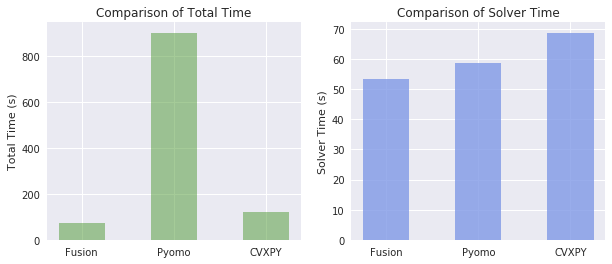## Discussion¶

Apparently, Fusion passes the model data to Mosek faster than the other ones. CVXPY is close to Fusion but its solver time is longer, mainly due to presolve (for example CVXPY enters variable bounds as constraints). In terms of total time Pyomo is behind the others because all the transformations are made in Python, as opposed to Fusion and CVXPY which call a C library. However, this is a huge model with 31 thousand constraints and 12 million variables and the difference will not be that big for normal-sized models.

On the other hand, Fusion and CVXPY allow you to express model in vectorized form (using matrix, vector notation). Pyomo is mainly based on sum expressions that can be defined by rule functions which makes modelling much easier. Therefore the time and effort spent on constructing the model in Pyomo was much smaller than with the other languages.

The decision of which one to use depends on the problem and the preferences of the user.

## CVXPY and Fusion on huge models¶

$\quad$The plots above show the performance of the modeling languages for 20 images. It is also reasonable to test the behaivor of solving times as the model gets larger and larger. In order to make this test same problem is solved with 50 images by using CVXPY and Fusion.

In :
n = 50
train_1 = ones[:n]
cvxpy_model.reset()
fusion_model.reset()


### CVXPY¶

In :
res_cvx = cvxpy_model.Wasserstein_BaryCenter(train_1)
print('\nTime Spent to solve problem with CVXPY: \n {0}'.format(cvxpy_model.time))
print('Time Spent in solver: \n {0}'.format(cvxpy_model.prob.solver_stats.solve_time))
print('The average Wasserstein distance between digits and the barycenter: \n {0}'.format(cvxpy_model.result))

Problem
Name                   :
Objective sense        : min
Type                   : LO (linear optimization problem)
Constraints            : 30812084
Cones                  : 0
Scalar variables       : 30733634
Matrix variables       : 0
Integer variables      : 0

Optimizer started.
Presolve started.
Linear dependency checker started.
Linear dependency checker terminated.
Eliminator started.
Freed constraints in eliminator : 0
Eliminator terminated.
Eliminator - tries                  : 1                 time                   : 0.00
Lin. dep.  - tries                  : 1                 time                   : 6.01
Lin. dep.  - number                 : 49
Presolve terminated. Time: 37.73
GP based matrix reordering started.
GP based matrix reordering terminated.
Problem
Name                   :
Objective sense        : min
Type                   : LO (linear optimization problem)
Constraints            : 30812084
Cones                  : 0
Scalar variables       : 30733634
Matrix variables       : 0
Integer variables      : 0

Optimizer  - solved problem         : the primal
Optimizer  - Constraints            : 43692
Optimizer  - Cones                  : 0
Optimizer  - Scalar variables       : 3560928           conic                  : 0
Optimizer  - Semi-definite variables: 0                 scalarized             : 0
Factor     - setup time             : 152.40            dense det. time        : 0.58
Factor     - ML order time          : 77.89             GP order time          : 70.64
Factor     - nonzeros before factor : 4.53e+06          after factor           : 9.72e+07
Factor     - dense dim.             : 0                 flops                  : 3.04e+11
Factor     - GP saved nzs           : 3.57e+07          GP saved flops         : 5.09e+11
ITE PFEAS    DFEAS    GFEAS    PRSTATUS   POBJ              DOBJ              MU       TIME
0   3.8e+03  1.0e+02  5.9e+07  0.00e+00   1.207747296e+07   0.000000000e+00   2.4e+01  195.47
1   9.7e-01  2.6e-02  1.5e+04  -1.00e+00  1.138202629e+07   -1.932435859e+05  6.1e-03  199.68
2   1.7e-01  4.7e-03  2.7e+03  4.69e+01   2.749170572e+04   -2.705073018e+03  1.1e-03  206.20
3   1.2e-01  3.3e-03  1.9e+03  8.18e+00   4.793007222e+03   -5.773295566e+02  7.6e-04  211.01
4   1.1e-01  3.1e-03  1.7e+03  3.34e+00   3.771396588e+03   -4.640287740e+02  7.1e-04  214.99
5   1.1e-01  2.9e-03  1.6e+03  3.09e+00   3.173273039e+03   -3.951025274e+02  6.7e-04  219.11
6   9.7e-02  2.6e-03  1.5e+03  2.94e+00   2.470985631e+03   -3.117937667e+02  6.1e-04  223.02
7   6.5e-02  1.8e-03  1.0e+03  2.74e+00   1.021523834e+03   -1.260039800e+02  4.1e-04  227.61
8   8.4e-03  2.3e-04  1.3e+02  2.13e+00   8.372742526e+01   -2.798064226e+00  5.2e-05  234.39
9   4.0e-03  1.1e-04  6.1e+01  1.18e+00   3.888935256e+01   -6.475636806e-01  2.5e-05  240.88
10  2.0e-03  5.4e-05  3.1e+01  1.09e+00   2.102550501e+01   1.461108737e+00   1.2e-05  245.43
11  1.6e-03  4.5e-05  2.5e+01  1.04e+00   1.781785581e+01   1.824394084e+00   1.0e-05  249.44
12  1.4e-03  4.0e-05  2.2e+01  1.03e+00   1.560289396e+01   1.976506458e+00   8.7e-06  253.43
13  1.2e-03  3.1e-05  1.9e+01  1.03e+00   1.404619009e+01   2.298815530e+00   7.5e-06  257.53
14  1.1e-03  2.8e-05  1.7e+01  1.02e+00   1.287129495e+01   2.405788065e+00   6.7e-06  261.85
15  8.5e-04  2.2e-05  1.3e+01  1.02e+00   1.082240990e+01   2.583882531e+00   5.3e-06  267.28
16  7.5e-04  1.9e-05  1.1e+01  1.01e+00   9.871522333e+00   2.665118594e+00   4.6e-06  271.43
17  6.3e-04  1.6e-05  9.6e+00  1.01e+00   8.789904345e+00   2.753524954e+00   3.9e-06  275.72
18  2.6e-04  6.0e-06  3.9e+00  1.01e+00   5.486142029e+00   3.037439240e+00   1.6e-06  280.57
19  2.1e-04  4.8e-06  3.2e+00  1.00e+00   5.049911898e+00   3.067269696e+00   1.3e-06  285.09
20  1.1e-04  2.8e-06  1.7e+00  1.00e+00   4.192997697e+00   3.120001209e+00   6.9e-07  289.72
21  8.3e-05  2.1e-06  1.3e+00  1.00e+00   3.937217141e+00   3.136827758e+00   5.2e-07  293.98
22  4.3e-05  1.4e-06  6.7e-01  1.00e+00   3.575235178e+00   3.153092018e+00   2.7e-07  298.06
23  2.6e-05  8.4e-07  4.0e-01  1.00e+00   3.417790137e+00   3.165912215e+00   1.6e-07  302.48
24  1.3e-05  4.4e-07  2.1e-01  1.00e+00   3.306492542e+00   3.174555301e+00   8.5e-08  306.79
25  5.5e-06  1.8e-07  8.5e-02  1.00e+00   3.233672573e+00   3.179897390e+00   3.5e-08  311.42
26  7.7e-07  1.9e-08  1.2e-02  1.00e+00   3.190109552e+00   3.182620943e+00   4.8e-09  316.45
27  3.3e-07  8.2e-09  5.1e-03  1.00e+00   3.185934904e+00   3.182730244e+00   2.1e-09  320.91
28  2.7e-08  6.7e-10  4.1e-04  1.00e+00   3.183064915e+00   3.182803646e+00   1.7e-10  326.93
29  6.5e-10  1.5e-11  9.3e-06  1.00e+00   3.182810905e+00   3.182805007e+00   3.8e-12  331.90
30  3.4e-11  1.4e-13  3.8e-08  1.00e+00   3.182805061e+00   3.182805037e+00   1.5e-14  335.98
Basis identification started.
Basis identification terminated. Time: 1.86
Optimizer terminated. Time: 361.28

Interior-point solution summary
Problem status  : PRIMAL_AND_DUAL_FEASIBLE
Solution status : OPTIMAL
Primal.  obj: 3.1828050614e+00    nrm: 2e+01    Viol.  con: 1e-10    var: 0e+00
Dual.    obj: 3.1828050373e+00    nrm: 2e+02    Viol.  con: 4e-16    var: 9e-14

Basic solution summary
Problem status  : PRIMAL_AND_DUAL_FEASIBLE
Solution status : OPTIMAL
Primal.  obj: 3.1828050375e+00    nrm: 2e+01    Viol.  con: 8e-07    var: 0e+00
Dual.    obj: 3.1828050375e+00    nrm: 2e+02    Viol.  con: 7e-15    var: 9e-14

Time Spent to solve problem with CVXPY:
512.632221698761
Time Spent in solver:
361.275288105011
The average Wasserstein distance between digits and the barycenter:
3.182805061402046

In :
plt.style.use("default")
plt.figure(figsize=(3.3,3.3))
plt.imshow(np.reshape(res_cvx.squeeze(), (28,28)))
plt.title('Barycenter')
plt.show()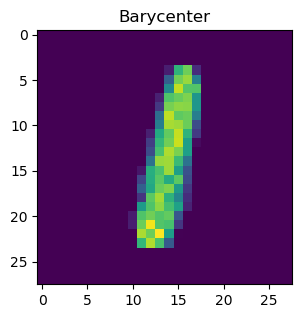### Fusion¶

In :
res_f = fusion_model.Wasserstein_BaryCenter(train_1)
print('\nTime Spent to solve problem with Fusion: \n {0}'.format(fusion_model.time))
print('Time Spent in solver: \n {0}'.format(fusion_model.M.getSolverDoubleInfo("optimizerTime")))
print('The average Wasserstein distance between digits and the barycenter: \n {0}'.format(fusion_model.objective))

Problem
Name                   : Wasserstein
Objective sense        : min
Type                   : LO (linear optimization problem)
Constraints            : 78400
Cones                  : 0
Scalar variables       : 30733585
Matrix variables       : 0
Integer variables      : 0

Optimizer started.
Presolve started.
Linear dependency checker started.
Linear dependency checker terminated.
Eliminator started.
Freed constraints in eliminator : 0
Eliminator terminated.
Eliminator - tries                  : 1                 time                   : 0.00
Lin. dep.  - tries                  : 1                 time                   : 0.89
Lin. dep.  - number                 : 49
Presolve terminated. Time: 13.31
GP based matrix reordering started.
GP based matrix reordering terminated.
Problem
Name                   : Wasserstein
Objective sense        : min
Type                   : LO (linear optimization problem)
Constraints            : 78400
Cones                  : 0
Scalar variables       : 30733585
Matrix variables       : 0
Integer variables      : 0

Optimizer  - solved problem         : the primal
Optimizer  - Constraints            : 43692
Optimizer  - Cones                  : 0
Optimizer  - Scalar variables       : 3560928           conic                  : 0
Optimizer  - Semi-definite variables: 0                 scalarized             : 0
Factor     - setup time             : 148.91            dense det. time        : 0.60
Factor     - ML order time          : 77.43             GP order time          : 67.30
Factor     - nonzeros before factor : 4.53e+06          after factor           : 9.72e+07
Factor     - dense dim.             : 0                 flops                  : 3.04e+11
Factor     - GP saved nzs           : 3.57e+07          GP saved flops         : 5.09e+11
ITE PFEAS    DFEAS    GFEAS    PRSTATUS   POBJ              DOBJ              MU       TIME
0   3.8e+03  1.0e+02  5.9e+07  0.00e+00   1.207747296e+07   0.000000000e+00   2.4e+01  166.33
1   9.7e-01  2.6e-02  1.5e+04  -1.00e+00  1.138202628e+07   -1.932435836e+05  6.1e-03  170.72
2   1.7e-01  4.7e-03  2.7e+03  4.69e+01   2.749170586e+04   -2.705073033e+03  1.1e-03  176.96
3   1.2e-01  3.3e-03  1.9e+03  8.18e+00   4.793007234e+03   -5.773295562e+02  7.6e-04  181.92
4   1.1e-01  3.1e-03  1.7e+03  3.34e+00   3.771396617e+03   -4.640287759e+02  7.1e-04  186.01
5   1.1e-01  2.9e-03  1.6e+03  3.09e+00   3.173273061e+03   -3.951025289e+02  6.7e-04  189.91
6   9.7e-02  2.6e-03  1.5e+03  2.94e+00   2.470985651e+03   -3.117937685e+02  6.1e-04  193.88
7   6.5e-02  1.8e-03  1.0e+03  2.74e+00   1.021523849e+03   -1.260039818e+02  4.1e-04  198.83
8   8.4e-03  2.3e-04  1.3e+02  2.13e+00   8.372815147e+01   -2.798139621e+00  5.2e-05  205.42
9   4.0e-03  1.1e-04  6.1e+01  1.18e+00   3.888892297e+01   -6.475319201e-01  2.5e-05  211.68
10  2.0e-03  5.4e-05  3.1e+01  1.09e+00   2.102558681e+01   1.461095266e+00   1.2e-05  216.47
11  1.6e-03  4.5e-05  2.5e+01  1.04e+00   1.781792814e+01   1.824383152e+00   1.0e-05  221.00
12  1.4e-03  4.0e-05  2.2e+01  1.03e+00   1.560302313e+01   1.976495303e+00   8.7e-06  225.04
13  1.2e-03  3.1e-05  1.9e+01  1.03e+00   1.404624228e+01   2.298817130e+00   7.5e-06  228.87
14  1.1e-03  2.8e-05  1.7e+01  1.02e+00   1.287134161e+01   2.405789410e+00   6.7e-06  233.13
15  8.5e-04  2.2e-05  1.3e+01  1.02e+00   1.082241807e+01   2.583885971e+00   5.3e-06  238.64
16  7.5e-04  1.9e-05  1.1e+01  1.01e+00   9.871538265e+00   2.665120797e+00   4.6e-06  242.84
17  6.3e-04  1.6e-05  9.6e+00  1.01e+00   8.789936046e+00   2.753525214e+00   3.9e-06  247.20
18  2.6e-04  6.0e-06  3.9e+00  1.01e+00   5.486120490e+00   3.037438811e+00   1.6e-06  252.18
19  2.1e-04  4.8e-06  3.2e+00  1.00e+00   5.049889518e+00   3.067269697e+00   1.3e-06  256.42
20  1.1e-04  2.8e-06  1.7e+00  1.00e+00   4.193063274e+00   3.120006971e+00   6.9e-07  261.06
21  8.3e-05  2.1e-06  1.3e+00  1.00e+00   3.937318985e+00   3.136828389e+00   5.2e-07  265.26
22  4.3e-05  1.4e-06  6.7e-01  1.00e+00   3.575228557e+00   3.153094207e+00   2.7e-07  269.78
23  2.6e-05  8.4e-07  4.0e-01  1.00e+00   3.417767887e+00   3.165915025e+00   1.6e-07  274.64
24  1.3e-05  4.4e-07  2.1e-01  1.00e+00   3.306549094e+00   3.174551508e+00   8.5e-08  278.83
25  5.5e-06  1.8e-07  8.5e-02  1.00e+00   3.233604776e+00   3.179902683e+00   3.5e-08  283.63
26  7.7e-07  1.3e-08  1.2e-02  1.00e+00   3.190062404e+00   3.182728989e+00   4.7e-09  289.08
27  3.4e-07  5.7e-09  5.1e-03  1.00e+00   3.186012714e+00   3.182772597e+00   2.1e-09  293.89
28  2.9e-08  4.9e-10  4.4e-04  1.00e+00   3.183083026e+00   3.182803490e+00   1.8e-10  299.39
29  1.1e-09  1.5e-11  1.6e-05  1.00e+00   3.182815207e+00   3.182804980e+00   6.6e-12  303.47
30  1.9e-11  1.7e-13  2.4e-08  1.00e+00   3.182805053e+00   3.182805037e+00   1.0e-14  307.66
31  4.4e-12  1.4e-13  2.1e-12  1.00e+00   3.182805038e+00   3.182805038e+00   1.3e-18  312.21
Basis identification started.
Basis identification terminated. Time: 1.87
Optimizer terminated. Time: 323.71

Interior-point solution summary
Problem status  : PRIMAL_AND_DUAL_FEASIBLE
Solution status : OPTIMAL
Primal.  obj: 3.1828050375e+00    nrm: 1e+00    Viol.  con: 7e-12    var: 0e+00
Dual.    obj: 3.1828050375e+00    nrm: 2e+02    Viol.  con: 0e+00    var: 9e-14

Basic solution summary
Problem status  : PRIMAL_AND_DUAL_FEASIBLE
Solution status : OPTIMAL
Primal.  obj: 3.1828050375e+00    nrm: 1e+00    Viol.  con: 4e-17    var: 8e-07
Dual.    obj: 3.1828050375e+00    nrm: 2e+02    Viol.  con: 0e+00    var: 7e-12

Time Spent to solve problem with Fusion:
369.8690595626831
Time Spent in solver:
323.7116389274597
The average Wasserstein distance between digits and the barycenter:
3.182805037502097

In :
plt.figure(figsize=(3.3,3.3))
plt.imshow(np.reshape(res_f,(28,28)))
plt.title('Barycenter')
plt.show()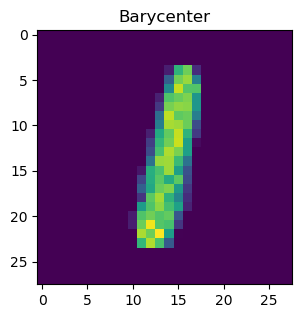In :
plt.figure(figsize=(8,3.5))

total_t50 = [fusion_model.time, cvxpy_model.time]
solver_t50 = [fusion_model.M.getSolverDoubleInfo("optimizerTime"), cvxpy_model.prob.solver_stats.solve_time]

#Total time plot
plt.subplot(1,2,1)
plt.bar(['Fusion', 'CVXPY'], height= total_t50,
width=0.4, color=(0.3, 0.6, 0.2, 0.5))
plt.ylabel("Total Time (s)")
plt.title("Comparison of Total Time")

#Solver time plot
plt.subplot(1,2,2)
plt.bar(['Fusion','CVXPY'], height=solver_t50,
width=0.4, color=(0.5, 0.6, 0.9, 0.8))
plt.ylabel("Solver Time (s)")
plt.title("Comparison of Solver Time")
plt.show()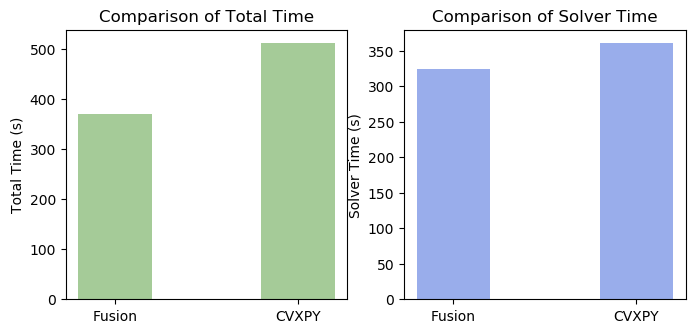This work is licensed under a Creative Commons Attribution 4.0 International License. The MOSEK logo and name are trademarks of Mosek ApS. The code is provided as-is. Compatibility with future release of MOSEK or the Fusion API are not guaranteed. For more information contact our support.